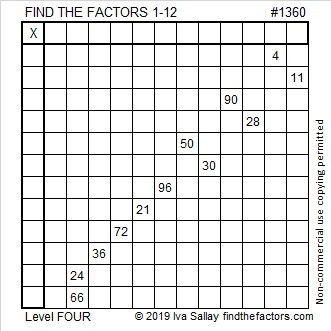# 1360 and Level 4

There is only one way to write the numbers 1 to 12 in the top row and the first column of this puzzle so that the puzzle could turn into a multiplication table. Can you find the solution?Print the puzzles or type the solution in this excel file: 12 Factors 1357-1365

Here are some facts about the number 1360:

• 1360 is a composite number.
• Prime factorization: 1360 = 2 × 2 × 2 × 2 × 5 × 17, which can be written 1360 = 2⁴ × 5 × 17
• 1360 has at least one exponent greater than 1 in its prime factorization so √1360 can be simplified. Taking the factor pair from the factor pair table below with the largest square number factor, we get √1360 = (√16)(√85) = 4√85
• The exponents in the prime factorization are 4, 1, and 1. Adding one to each exponent and multiplying we get (4 + 1)(1 + 1)(1 + 1) = 5 × 2 × 2 = 20. Therefore 1360 has exactly 20 factors.
• The factors of 1360 are outlined with their factor pair partners in the graphic below.1360 is the sum of two squares in two different ways:
36² + 8² = 1360
28² + 24² = 1360

1360 is the hypotenuse of FOUR Pythagorean triples:
208-1344-1360 which is 16 times (13-84-85)
576-1232-1360 which is 16 times (36-77-85)
640-1200-1360 which is (8-15-17) times 80
816-1088-1360 which is (3-4-5) times 272

This site uses Akismet to reduce spam. Learn how your comment data is processed.